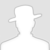FreeBuf.COM网络安全行业门户，每日发布专业的安全资讯、技术剖析。FreeBuf+小程序

【攻防演练】解决nmap无法批量扫描B段资产
2021-03-11 11:24:13

`a = int(input("请输入您要扫描的ip A段地址："))while not 0 < a <= 255:a = int(input("您的输入有误，请重新输入您要扫描的ip A段地址："))​b = int(input("请输入您要扫描的ip B段地址："))while not 0 <= b <= 255:b = int(input("您的输入有误，请重新输入您要扫描的ip B段地址："))​ipAB = str(a) + "." + str(b)while 1:c1 = int(input("请输入您要扫描的ip C段地址（起始地址）："))while not 0 <= c1 <= 255:c1 = int(input("您的输入有误，请重新输入您要扫描的ip C段地址（起始地址）："))​c2 = int(input("请输入您要扫描的ip C段地址（结束地址）："))while not 0 <= c2 <= 255:c2 = int(input("您的输入有误，请重新输入您要扫描的ip C段地址（结束地址）："))if c2 < c1:print("您的输入有误，青重新输入！")else:break​ip = []​g = input("请输入要您要储存的IP的方式：\n1.单条IP方式，例：192.168.0.1\n2.同一C段IP存储为/24形式，例：192.168.0.0/24\n")​while 1:if g=="1":for i in range(c2 + 1 - c1):e = c1 + ie = str(e)for j in range(256):j = str(j)ip.append(ipAB + "." + e + "." + j)breakelif g=="2":for i in range(c2 + 1 - c1):e = c1 + ie = str(e)ip.append(ipAB + "." + e + ".0/24")breakelse:g=input("您的输入有误，请重新输入要您要储存的IP的方式：\n1.单条IP方式，例：192.168.0.1\n2.同一C段IP存储为/24形式，例：192.168.0.0/24\n")​​​f = input("是否要保存全部ip地址？(Y/N)：").upper()while 1:if f == "Y":spath = input(r"请输入您要保存的路径+文件名，例如（C:\test.txt），只输入文件名则默认保存在工具路径下：")while 1:try:with open(spath, "a+") as file:for i in range(len(ip)):file.write(ip[i] + "\n")print("保存成功！")breakexcept:spath=input(r"您输入的路径路径有误，请重新输入您要保存的路径+文件名，例如（C:\test.txt），只输入文件名则默认保存在工具路径下：")breakelif f == "N":print("没有保存ip地址！")breakelse:f = input("您的输入有误，请重新输入(Y/N)：")`

# nmap # Python工具 # 资产扫描 # HW工具

+ 收入我的专辑
+ 加入我的收藏\
• 0 文章数
• 0 评论数
• 0 关注者